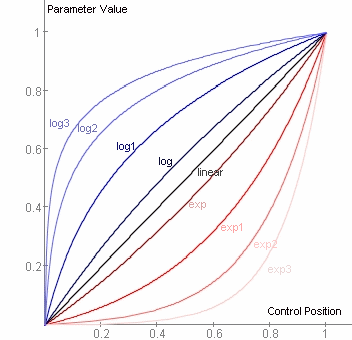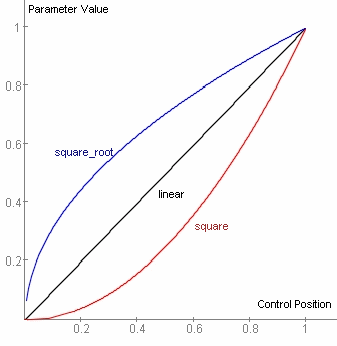KUIML Blue Cat's User Interface Programming LanguageTypes of Attributes

# All Attribute Types

The types of values used for attributes in KUIML are listed below:

Type Syntax Examples Comment

identifier

Any alphanumeric or '_' character, without space.

'myControl', 'slider1','param_slider'

'my Control' of 'my-control' are invalid identifiers.

string

Any character

Hello!

color

RGB hexadecimal value preceded by '#'

'#ffffff' for white, '#ff0000' for red.

This is the same syntax as HTML colors.

boolean

'true' or 'false'

real number

digits.digits

'5.3' '10' '3.02' '0.4'

The language only supports English notation with '.' for the delimiter.

parameter type

'real' or 'boolean', 'integer' or 'enumeration'

Real stands for real number in floating point notation (5.3)

number of pixels

Any positive integer value

145

percentage

Real number followed by ''

'20' '33.3'

position in pixels

Any integer value

-5

vertical alignment

'top' or 'bottom' or 'center'

horizontal alignment

'left' or 'right' or 'center'

orientation

'vertical' or 'horizontal'

'vertical'

graph style

'curve' or 'bargraph'

'bargraph'

The bargraph style displays bars from the origin of the graph to the value of the curve, whereas the curve style draws curve points connected by segments in the case of non continuous curves.

file path

Relative file path. The root of all paths is the skin file directory.

'xxx.bmp', or 'images/xxx.bmp'

image file path

See file path

Supported formats:

bmp (8,16 or 24 bits) or png files. For more information see the Images section.

cursor

'system::xxx' where xxx is a valid cursor type, or (Windows only) valid cursor file path.

System::hand or 'mycursor.cur'

event

Events are fired when something happens and can be used to trigger actions.

Events are exposed by the data model and can be used to perform specific actions when fired.

script

script code.

param id

see identifier

The identifier must point to an object of appropriate PARAM type.

curve id

see identifier

The identifier must point to an object of appropriate CURVE type.

surface id

see identifier

The identifier must point to an object of appropriate SURFACE type.

string id

see identifier

The identifier must point to an object of appropriate STRING type.

action id

see identifier

The identifier must point to an object of appropriate ACTION type.

group id

see identifier

The identifier must point to an object of appropriate GROUP type.

property id

see identifier

The identifier must point to an object of appropriate PROPERTY type.

string with param fields

String containing parameter fields enclosed into brackets

'the value is: {value} {unit}'

Valid fields are:

{value}, {unit}, {name}, {default}, {min}, {max}, {comment} and (V1.3.4) {text_value}

param value formatting

Flag|max_char|.digits (all fields are optional), where:

Flag values (can be mixed):

• '+' to show a '+' when the value is positive

• '0' : value is padded with 0s. Valid only if followed by the max_char field.

max_char is the maximum number of characters to use for the text (sign and '.' included)

'.' followed by digits: number of characters reserved for the decimal part

'+8.2' will show a number with its sign, with a maximum of 8 characters (including '.') and 2 characters reserved for the digits

'.0' will show a number without decimal part.

'+05.0' will show a value with 5 characters without any decimal part, padded with '0' if needed.

Using the max_char field avoids the text to move on the screen when the value changes if you used a fixed size font such as 'Courier New'.

For programmers, the syntax is very similar to the formatting options of 'printf' standard c function.

response curve

A value in this list: 'linear' 'log' 'exp' 'log1' 'exp1' 'log2' 'exp2' 'log3' 'exp3' 'square_root' 'square'

See the Response Curves graphes below for an overview of available curves.

# Response Curvesinfluence of the 'response_curve' attribute - exp & log influence of the 'response_curve' attribute - square & square_root

# Math Formula

The Language supports mathematical formulas for several purposes (attributes computation, curves or surfaces defined with formulas etc.). The syntax is similar to common mathematical notations on computer systems. Example:

```  3*x^2+cos(pi/2)*x+1
```

The language supports most common operators (*,/,+,-,^) as well as several predefined mathematical functions. The complete list is available below:

Symbol Operation Example Comment

+

x+1

*

Multiplication

x*y

-

Substraction

5-z

/

Division

x/2

^

Power

a^2 represents "a square"

%

Modulo

x%5 means "x modulo 5"

==

Equals

a==b means "a equals b" and the result is true or false

You can also use '='

!=

Not Equals

a!=b means "a does not equal b" and the result is true or false

You can also use '<>'

<

Lower than

a<b means "a stricly lower than b" and the result is true or false

>

Greater than

a>b means "a stricly greater than b" and the result is true or false

<=

Lower than or equal

a<=b means "a lower than or equal to b" and the result is true or false

>=

Greater than or equal

a>=b means "a greater than or equal to b" and the result is true or false

||

Boolean "OR"

a||b means "a or b"

You can also use 'or' (case sensitive).

&&

Boolean "AND"

a&&b means "a and b"

You can also use 'and' (case sensitive).

!

Boolean "NOT"

!(x==y) is equivalent to "x!=y" and means "x is not equal to y"

You can also use 'not' (case sensitive).

sqrt

Square root

sqrt(2)

abs

Absolute value

abs(-1) equals to 1

floor

Rounds to lowest integer

floor(1.25) equals to 1

ceil

Rounds to highest integer

ceil(1.25) equals to 2

round

Rounds to closest integer

round(1.25) equals to 1, and round(1.75) equals to 2

sin

sine

sin(pi)

cos

cosine

cos(x)

tan

tangent

tan(2*pi*x)

exp

Exponential

exp(10)

log

Logarithm

log(1) is equal to 0

sinh

Hyperbolic sine

sinh(pi)

cosh

Hyperbolic cosine

cosh(x)

tanh

Hyperbolic tangent

tanh(x)

pi

Pi Constant (3.14159...)

cos(pi) is equal to -1

min

Minimum

min(1,2) is equal to 1

max

Maximum

max(1,2) is equal to 2

bitvalue

Value of specfied bit number

bitvalue(x,n) returns the value of the nth bit of integer x

if

if(condition,then,else)

if(true,a,b) returns a and if(false,a,b) returns b